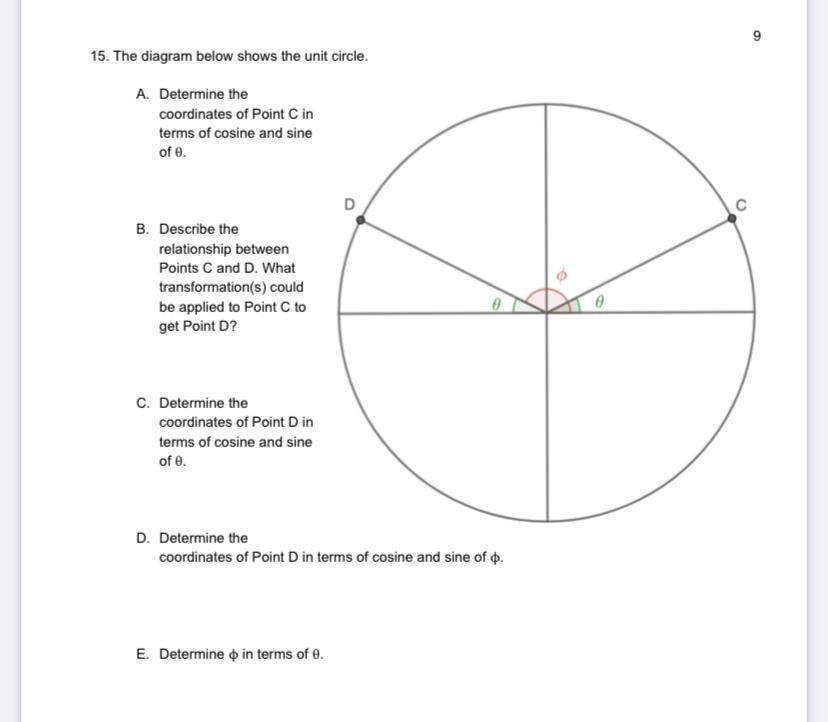Question:

# 15. The diagram below shows the unit circle. A. Determine the coordinates of Point C in terms of cosine and sine of e. C B. Desc15. The diagram below shows the unit circle. A. Determine the coordinates of Point C in terms of cosine and sine of e. C B. Describe the relationship between Points C and D. What transformation(s) could be applied to Point to get Point D? 0 C. Determine the coordinates of Point Din terms of cosine and sine of e. D. Determine the coordinates of Point D in terms of cosine and sine of o. E. Determine o in terms of e.